#### A uniform magnetic field B of 0.3 T is along the positive Z-direction.  A rectangular loop (abcd) of sides 10 cm×5 cm carries a current I of 12 A.  Out of the following different orientations which one corresponds to stable equilibrium ? Option 1)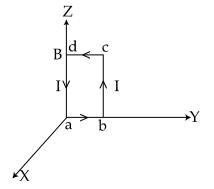Option 2)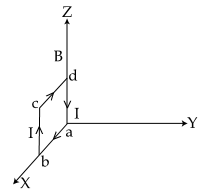Option 3)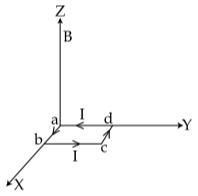Option 4)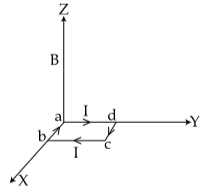As we learnt in

Magnetic moment (M) -

M=NiA

- wherein

N-number of turns in the coil

i-current throughout the coil

A-area of the coil

and

Current loop as a magnetic dipole -

A current carrying circular coil behaves as a bar magnet whose magnetic monent is M

- wherein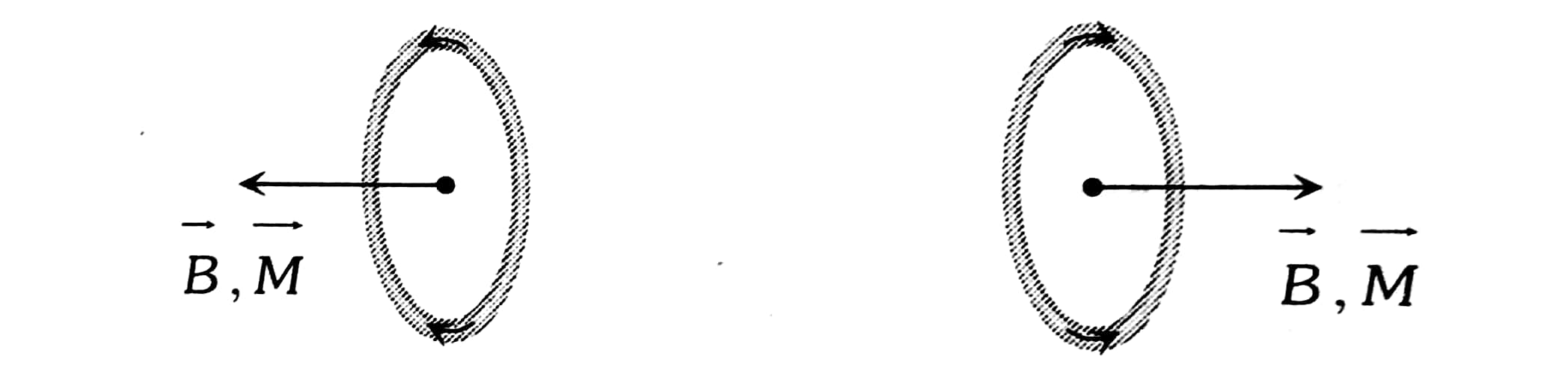Magnetic moment of current carrying rectangular loop of Area A is given by M= NiA

Magnetic moment of current carrying coil is a vector and its direction is given by right hand thumb rule,

For rectangular loop,at centre due to current in loop andare always parallel

Option 1)This is incorrect option.

Option 2)This is incorrect option.

Option 3)This is correct option.

Option 4)This is incorrect option.

#### divya.saini